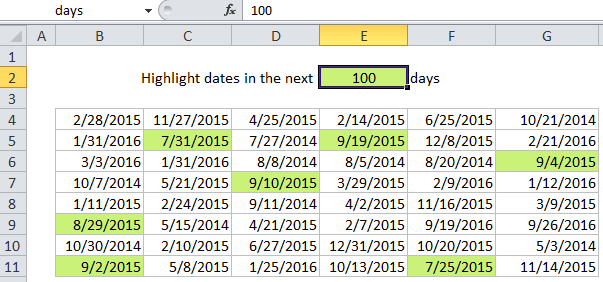# Highlight dates in the next N days in Excel

This tutorial shows how to Highlight dates in the next N days in Excel  using the example below;

## Formula

`=AND(A1>TODAY(),A1<=(TODAY()+days))`## Explanation

If you want to highlight dates that occur in the next N days with conditional formatting, you can do so with a formula that uses the TODAY function with AND. This is a great way to visually flag things like expiration dates, deadlines, upcoming events, and dates relative to the current date.

For example, if you have dates in the range B4:G11, and want to highlight cells that occur in the next 30 days, select the range and create a new CF rule that uses this formula:

`=AND(B4>TODAY(),B4<=(TODAY()+30))`

Note: it’s important that CF formulas be entered relative to the “active cell” in the selection, which is assumed to be B4 in this case.

Worked Example:   Conditional Formatting Rules in Excel

Once you save the rule, you’ll see the dates occurring in the next 30 days highlighted.

### How this formula works

The AND function takes multiple arguments and returns TRUE only when all arguments return TRUE. The TODAY function returns the current date. Dates in Excel are simply large serial numbers, so you can create a new relative date by adding or subtracting days. TODAY() + 30 creates a new date 30 days in the future, so when a days is greater than today and less than today + 30, both conditions are true, and the AND function returns true, triggering the rule.

Worked Example:   Excel Data validation only dates between

### Variable days

Of course, you can adjust days to any value you like:

```=AND(B4>TODAY(),B4<=(TODAY()+7)) // next 7 days
=AND(B4>TODAY(),B4<=(TODAY()+45)) // next 45 days```

### Use other cells for input

You don’t need to hard-code the dates into the rule. To make a more flexible rule, you can use other cells like variables in the formula. For example, you can name cell E2 “days” and rewrite the formula like so:

`=AND(B4>TODAY(),B4<=(TODAY()+days))`

When you change either date, the conditional formatting rule will respond instantly. By using other cells for input, and naming them as named ranges, you make the conditional formatting interactive and the formula is simpler and easier to read.

Worked Example:   Highlight values greater than in Excel